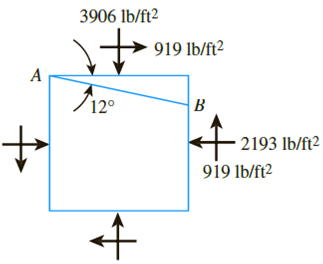Chapter 10, Problem 10.2PPrinciples of Geotechnical Enginee...

9th Edition
Braja M. Das + 1 other
ISBN: 9781305970939

Solutions

Chapter
SectionPrinciples of Geotechnical Enginee...

9th Edition
Braja M. Das + 1 other
ISBN: 9781305970939
Textbook Problem

Repeat Problem 10.1 for the element shown in Figure 10.35.10.1 A soil element is shown in Figure 10.34. Determine the following:a. Maximum and minimum principal stressesb. Normal and shear stresses on plane ABUse Eqs. (10.3), (10.4), (10.6), and (10.7).Figure 10.35

(a)

To determine

Calculate the maximum and minimum principal stresses.

Explanation

Given information:

The normal stress along x axis (σx) is 2,193lb/ft2.

The normal stress along y axis (σy) is 3,906lb/ft2.

The shear stress along xy axis (τxy) is 919lb/ft2.

The angle (θ) is 168°.

Calculation:

Calculate the maximum principal stress (σ1) using the relation.

σ1=σy+σx2+[σy+σx2]2+τxy2

Substitute 2,193lb/ft2 for σx, 3,906lb/ft2 for σy, and 919lb/ft2 for τxy.

σ1=3,906+2,1932+[3,9062,1932]2+9192=3,049.5+1,578,153.25=3,049.5+1,256.25=4,305

(b)

To determine

Calculate the normal and shear stresses on plane AB.

Still sussing out bartleby?

Check out a sample textbook solution.

See a sample solution

The Solution to Your Study Problems

Bartleby provides explanations to thousands of textbook problems written by our experts, many with advanced degrees!

Get Started

What is the Turing Test?

Fundamentals of Information Systems

What is RADIUS? What advantage does it have over TACACS?

Principles of Information Security (MindTap Course List)

Convert 10,000 ft-lbf of energy into BTU, Joules, and kilojoules.

Fundamentals of Chemical Engineering Thermodynamics (MindTap Course List)

Determine the magnitudes of the forces acting on the bracket at B and C.

International Edition---engineering Mechanics: Statics, 4th Edition

What are some types of keyboards?

Enhanced Discovering Computers 2017 (Shelly Cashman Series) (MindTap Course List)